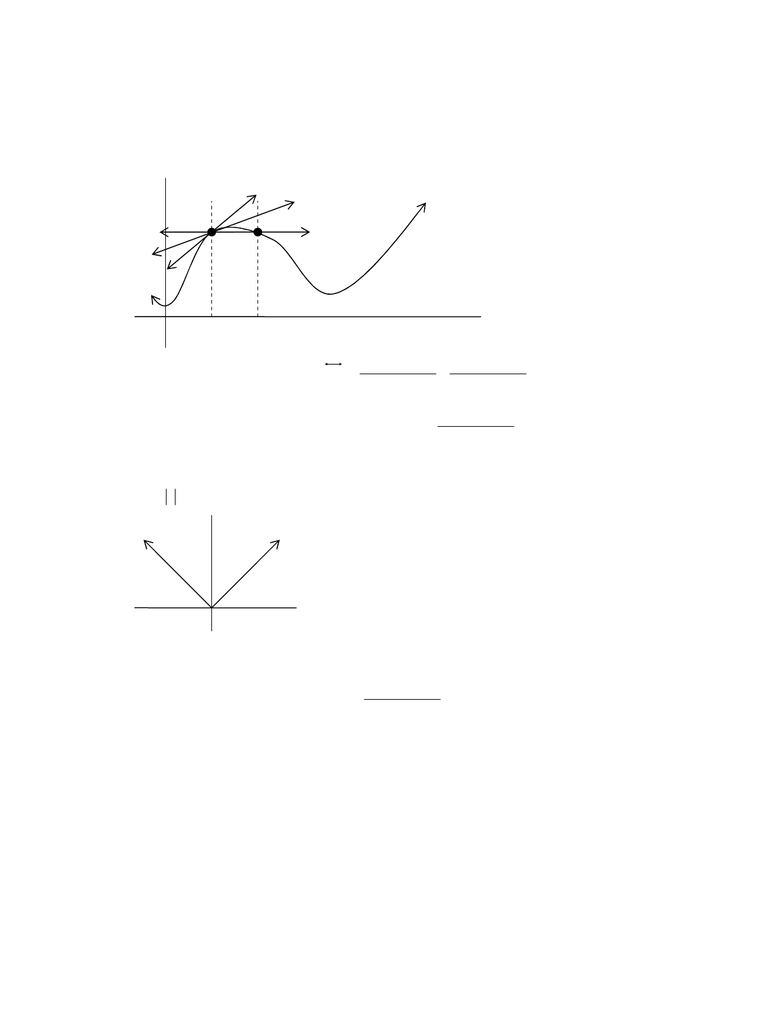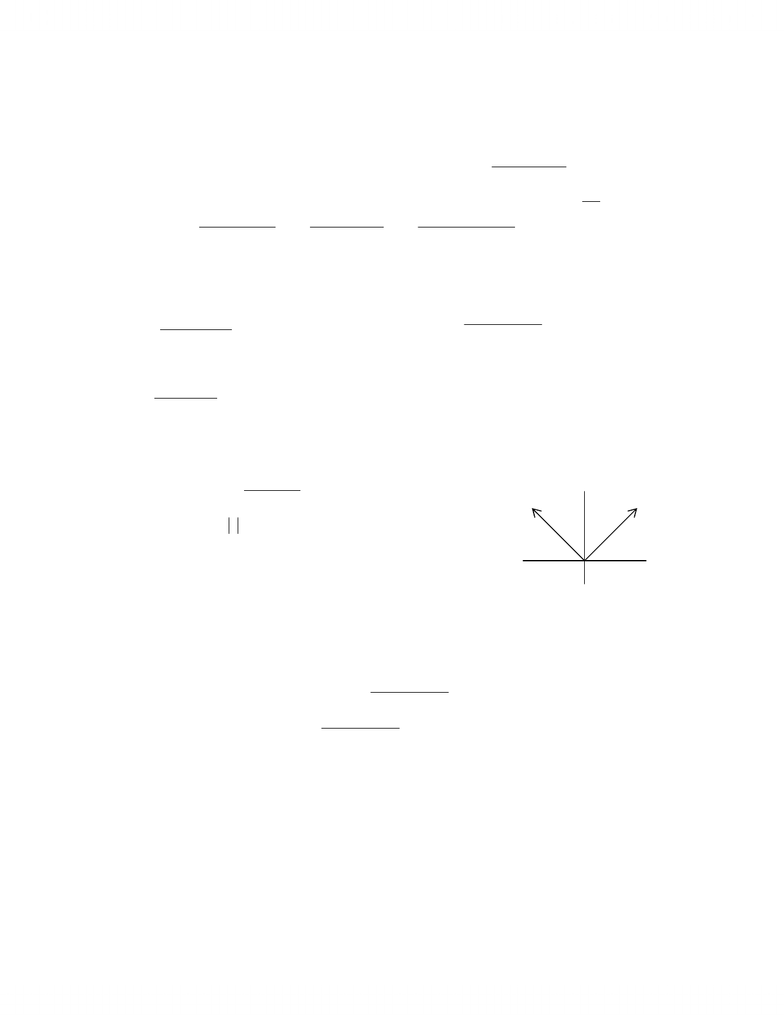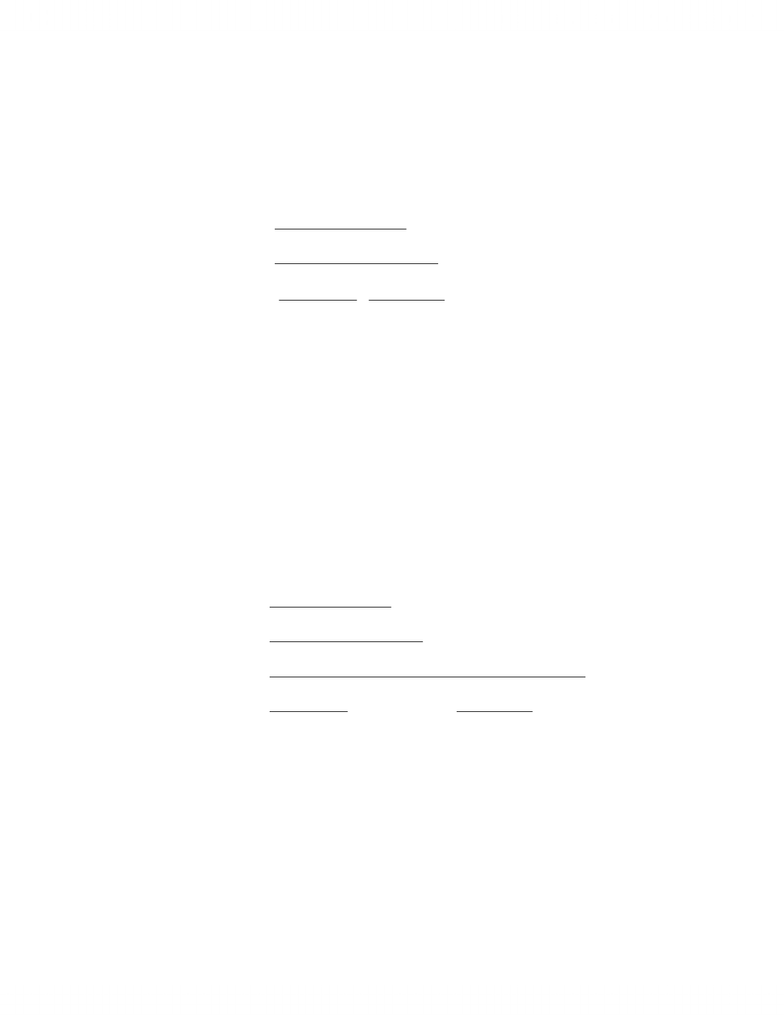# Summary notes 2

76 views38 pages
School
Department
Course
ProfessorMAT137Y1b.doc
Page 1 of 38
Lecture #12 Thursday, October 16, 2003
DIFFERENTIATION
What is the slope of the secant line PQ?
(
)
(
)
( )
(
)
(
)
h
cfhcf
chc
cfhcf+
=
+
+ .
Idea: Get the slope of the tangent line as a limit of slopes of secant lines.
The slope of the tangent line at
c
x
=
ought to be
(
)
(
)
h
cfhcf
h
+
0
lim.
Example
(
)
xxf= at 0
=
x
This DOESNT have a well defined tangent line
Definition
f is differentiable at
c
x
=
if the limit
(
)
(
)
h
cfhcf
h
+
0
lim exists. If it does, we call it the derivative of f at
c and we denote it by
(
)
cf
.
Geometrically
(
)
cf
is the slope of the tangent line going through
(
)
(
)
cfc,.
What is the equation for tangent line?
(
)
(
)
(
)
cxcfcfy
=
P
c
Q
f
(
)
(
)
hcfhc++ ,
(
)
(
)
cfc,
c + h
www.notesolution.com
Unlock document

This preview shows pages 1-3 of the document.
Unlock all 38 pages and 3 million more documents.MAT137Y1b.doc
Page 2 of 38
Lecture #13 Tuesday, October 21, 2003
Example
For function
(
)
2
xxf=, the derivative of f at 2
=
c is
( )
(
)
(
)
( )
44lim
22
lim2
0
22
0
=+=
+
=
h
h
h
f
hh
.
The derivative of f is itself a function for
(
)
2
xxf= repeat the same calculation for any value of c.
( )
(
)
(
)
(
)
(
)
( )
chc
h
chchc
h
chc
h
cfhcf
cf
hhhh
22lim
2
limlimlim
0
222
0
22
00
=+=
++
=
+
=
+
=
At any fixed value of x,
(
)
xxf2=
.
Definition
The derivative of f is a function, denoted f
, and
( )
(
)
(
)
h
xfhxf
xf
h
+
=
0
lim, if it exists.
Terminology
To differentiate a function is to find the derivative.
Notice: The function f has to be defined in the interval
(
)
δ+δxx , in order for
(
)
xf
to be defined.
Example
Actually, even if f is continuous on
(
)
δ+δxx ,, it doesnt mean
(
)
xf
is defined.
Consider
(
)
0,== cxxf
Theorem
If f is differentiable at x, the f is continuous.
Being differentiable is better’ than being continuous.
Proof:
Because f is differentiable at x,
(
)
(
)
( )
xf
h
xfhxf
h
=
+
0
lim
( ) ( )( )
(
)
(
)
( )
00limlim
00
=
=
+
=+ xfh
h
xfhxf
xfhxf
hh
So f is continuous.
DIFFERENTIATION RULES
Building Blocks
If
(
)
cxf= (a constant function), then
(
)
0=
xf for all x.
If
(
)
xxf=, then
(
)
1=
xf for all x.
f(x)
x
www.notesolution.com
Unlock document

This preview shows pages 1-3 of the document.
Unlock all 38 pages and 3 million more documents.MAT137Y1b.doc
Page 3 of 38
Theorem: Sums and Scalar Multiples
Let f, g be differentiable at x and α a constant.
Then
(
)
gf+ and fα are differentiable, then
( ) ( ) ( ) ( )
xgxfxgf
+
=
+
( ) ( ) ( )
xfxf
α=
α
Proof:
( ) ( )
(
)
(
)
(
)
(
)
( ) ( ) ( ) ( )
( ) ( ) ( ) ( )
( ) ( )
xgxf
h
xghxg
h
xfhxf
h
xgxfhxghxf
h
xgfhxgf
xgf
h
h
h
+
=
+
+
+
=
+++
=
+
+
+
=
+
0
0
0
lim
lim
lim
Example
If
(
)
xxf10=,
(
)
10=
xf
Theorem: Differences and Linear Combinations
From the Sums and Scalar Multiples rule,
( ) ( ) ( ) ( )
xgxfxgf
=
( ) ( ) ( ) ( ) ( )
xfxfxfxfff nnnn
α++
α+
α=
α++α+α...... 22112211
Theorem: Product Rule
If f and g are differentiable at x, then gf is differentiable and
( ) ( ) ( ) ( ) ( ) ( )
xgxfxgxfxgf
+
=
Proof:
( ) ( )
(
)
(
)
(
)
(
)
( ) ( ) ( ) ( )
( ) ( ) ( ) ( ) ( ) ( ) ( ) ( )
( ) ( ) ( ) ( ) ( ) ( )
( ) ( ) ( ) ( )
xgxfxgxf
gf
h
xghxg
xfhxg
h
xfhxf
h
xgxfhxgxfhxgxfhxghxf
h
xgxfhxghxf
h
xgfhxgf
xgf
hh
h
h
h
+
=
+
++
+
=
+++++
=
++
=
+
=
.continuous are they able,differenti are , Because
limlim
lim
lim
lim
00
0
0
0
Theorem: Power Rule
Using the Product Rule, we derive the Power Rule.
For Z
>
nn ,0, if
(
)
n
xxf=, then
(
)
1
=
n
nxxf.
Proof (by induction):
True for 1=k
Assume
(
)
1
=
kk kxx . Prove for 1+k:
www.notesolution.com
Unlock document

This preview shows pages 1-3 of the document.
Unlock all 38 pages and 3 million more documents.

## Get access